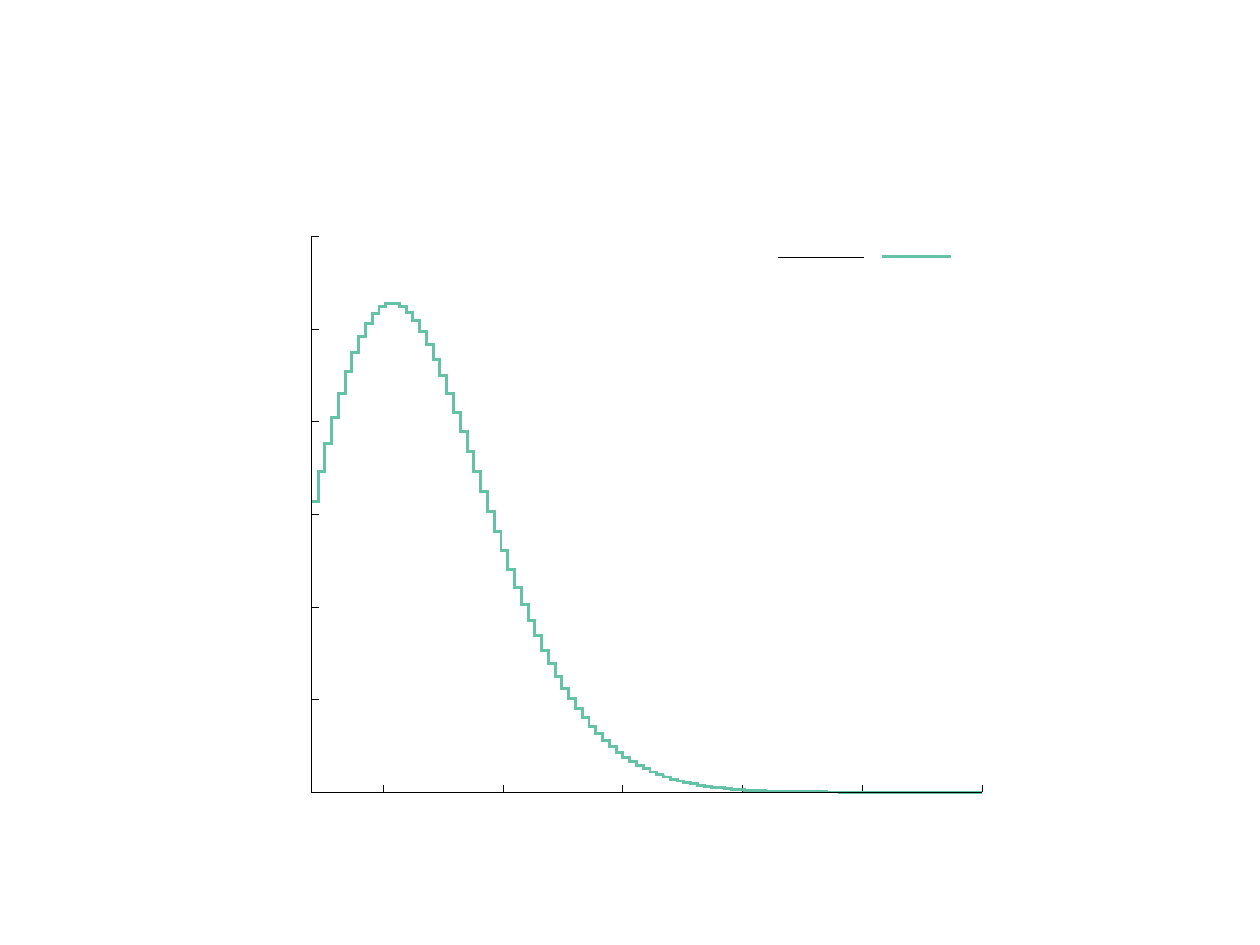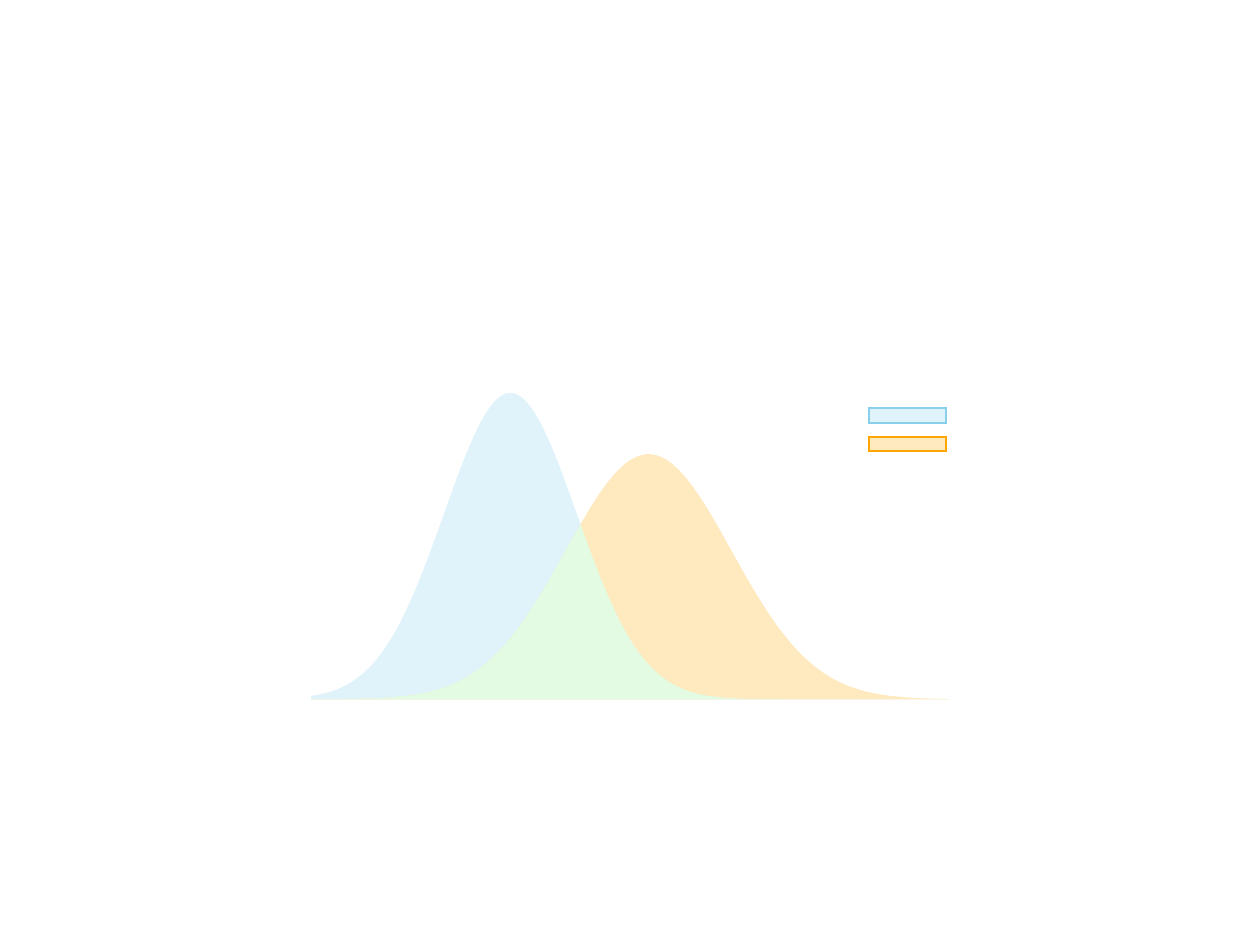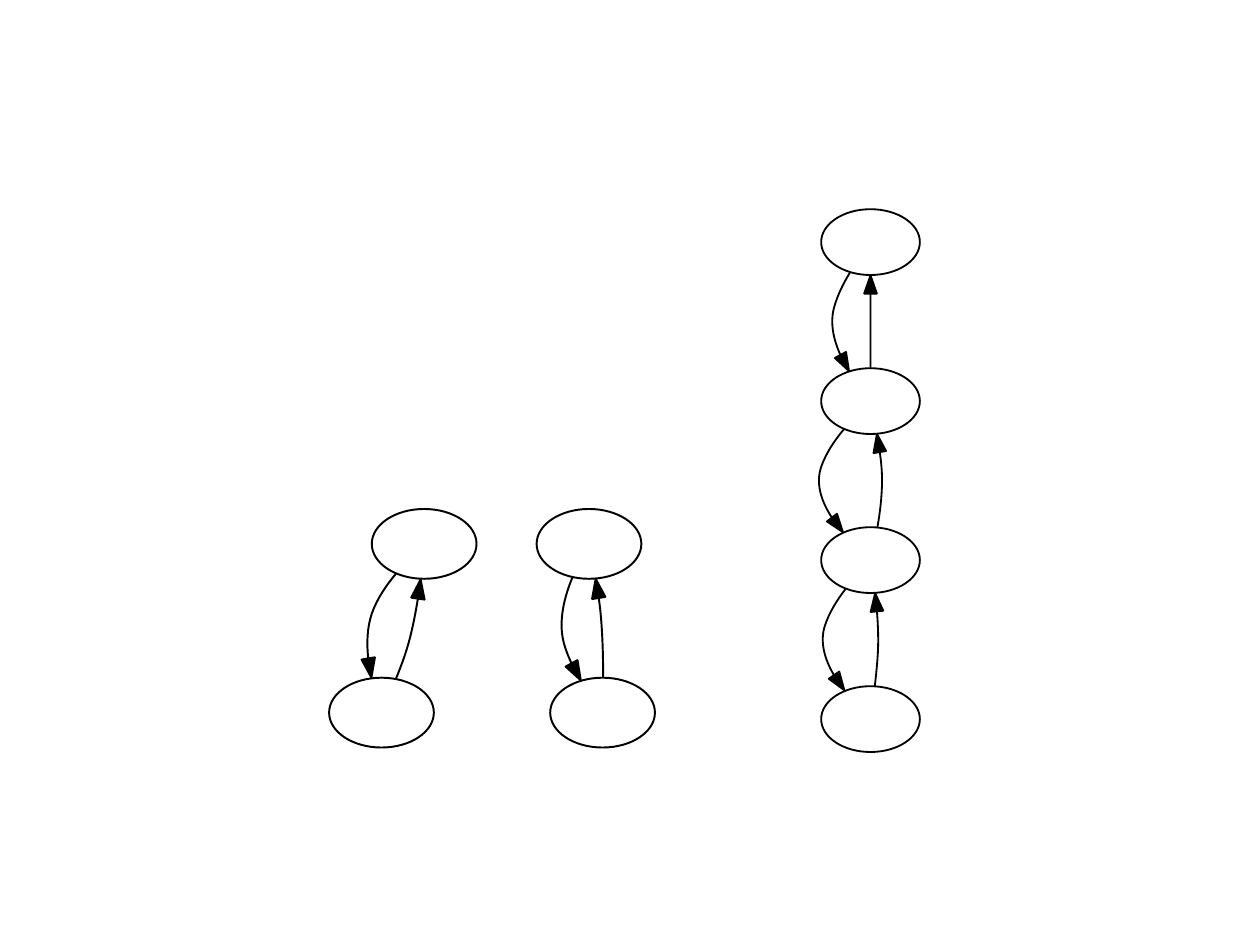The Monte Carlo method, Markov chains,
and the Metropolis algorithm
Juan M. Bello-Rivas
jmb@ices.utexas.eduOutline
1 High-dimensional integration
2 Markov chains
3 Metropolis algorithmOutline
1 High-dimensional integration
2 Markov chains
3 Metropolis algorithmHigh-dimensional integration
Suppose we have
Z
X
f(x) π(x) dx
where X R
n
, f : X R, and π : X R
0
is a probability
density function.High-dimensional integration
In 1D, if X = (a, b) R, then divide X into I N subintervals.
Then,
Z
X
f(x) π(x) dx =
I
X
i=1
w
i
f(x
i
)π(x
i
) + O(I
r
)
as I with r N.
Problem: If X = (a, b)
n
, then the number of nodes grows with n
as I
n
.High-dimensional integration
Another problem: curse of dimensionality.
0
1
2
3
4
5
6
5 10 15 20 25 30
Volume V (n)
Dimension n
n-dimensional volume: V (n) =
π
n/2
Γ(n/2+1)High-dimensional integration
Solution: Monte Carlo integration.
Z
X
f(x) π(x) dx = E
π
[f(x)]
and
E
π
[f(x)] =
1
I
I
X
i=1
f(x
i
) + O(I
1/2
)
as I , where the x
i
are random samples from the
probability distribution with density π.
The error term is bad but it is independent of n. Also note that
method is independent of the smoothness of the integrand.Outline
1 High-dimensional integration
2 Markov chains
3 Metropolis algorithmDeﬁnition
Let X be a ﬁnite set called state space. A Markov chain is a real
matrix A(x, y) such that
1 A(x, y) 0 y X and
2
P
y∈X
A(x, y) = 1
for each x X .Deﬁnition
We can regard each row in A(x, y) as a probability mass
function and then consider a random walk directed by the
following procedure:
1 from state x choose a new state y with probability A(x, y),
2 from state y choose a new state z with probability A(y, z),
3 and so on.
The outcomes X
0
= x, X
1
= y, X
2
= z, . . . are referred to as a
run of the chain starting at x.
Remark
In other words, the value of A(x, y) completely determines the
conditional probability of reaching y from x.Example
Let p (0, 1) and X = {R, I, P } with
R = 1, I = 2, P = 3. We can think of the Markov
chain
A =
0 1 0
1 p 0 p
1 0 0
as the graph on the right.
R
I
1 1 - p
P
p
1From the previous deﬁnitions and the chain rule
1
of conditional
probability, we know that
P(X
1
= y | X
0
= x) = A(x, y)
and
P(X
2
= z, X
1
= y | X
0
= x)
= P(X
2
= z | X
1
= y, X
0
= x) P(X
1
= y | X
0
= x)
= P(X
2
= z | X
1
= y) P(X
1
= y | X
0
= x)
= A(y, z) A(x, y).
1
P(A, B) = P(A|B) P(B).Thus, since
P(X
2
= z, X
1
= y | X
0
= x) = A(y, z) A(x, y),
we conclude that
P(X
2
= z | X
0
= x) =
X
y∈X
P(X
2
= z, X
1
= y | X
0
= x)
=
X
y∈X
A(x, y)A(y, z)
= A
(2)
(x, z).
This leads us to the expression of the n-th step Markov chain:
A
(n)
(x, y) = P(X
n
= y | X
0
= x).Deﬁnition
A Markov chain A is said to be irreducible if for all x, y X
there exists n N such that A
(n)
(x, y) > 0.
Deﬁnition
An irreducible Markov chain is aperiodic if
gcd({n N | A
(n)
(x, x) > 0}) = 1
for some x X .Example
The Markov chain given by
1/2 1/2
1/2 1/2
is aperiodic whereas the Markov chain given by
0 1
1 0
is periodic with period two. Both chains are irreducible.Deﬁnition
Let A(x, y) be a Markov chain over the state space X . A
stationary distribution of A is given by a probability mass
function π(x) such that
π(x) 0 for each x X (1)
and
π(x) =
X
y∈X
π(y)A(y, x) (2)Remark
Note that condition (2) can be interpreted as saying that A(x, y)
has a left eigenvector with corresponding eigenvalue equal to 1
that coincides with the probability mass function π(x).
This suggests a way of sampling from π(x).Deﬁnition
Let µ and ν be two probability mass functions over the same state
space X . The total variation distance between µ and ν is
deﬁned as
kµ νk
TV
= max
A⊂X
|µ(A) ν(A)|
µ
ν
1
2
3
Interpretation of TV distance.Theorem (Fundamental theorem of Markov chains)
If A is an irreducible Markov chain, then there exists a unique
stationary distribution π of A. Moreover, if A is aperiodic, then for
all x X
lim
n→∞
A
(n)
(x, ·) = π(·) in k · k
TVEﬀect of break-down of irreducibility
Let X = {1, 2, 3, 4}. Consider the family of matrices given by
A
t
=
0 1 0 0
1 t/2 0 t/2 0
0 t/2 0 1 t/2
0 0 1 0
,
where 0 t 1. Clearly,
A
0
=
0 1 0 0
1 0 0 0
0 0 0 1
0 0 1 0
and A
1
=
0 1 0 0
1/2 0 1/2 0
0 1/2 0 1/2
0 0 1 0
.Eﬀect of break-down of irreducibility
1
2
1 1
3
4
1 1
(a) A
0
1
2
1 1/2
3
1/2 1/2
4
1/2 1
(b) A
1Eﬀect of break-down of irreducibility
We can characterize the invariant subspaces of A
T
t
. Indeed, the
eigenvalues are
{1, 1 t/2, 1 + t/2, 1} ,
and the corresponding eigenspaces are spanned by the vectors
E
1
= (1, 2/(2 t), 2/(2 t), 1),
E
1t/2
= (1, 1, 1, 1),
E
1+t/2
= (1, 1, 1, 1),
E
1
= (1, 2/(2 t), 2/(2 t), 1).
The negative and positive parts of E
1t/2
when t = 0 allow us to
identify metastable states.Outline
1 High-dimensional integration
2 Markov chains
3 Metropolis algorithm